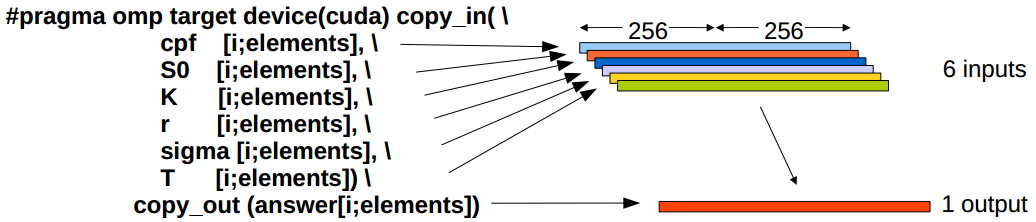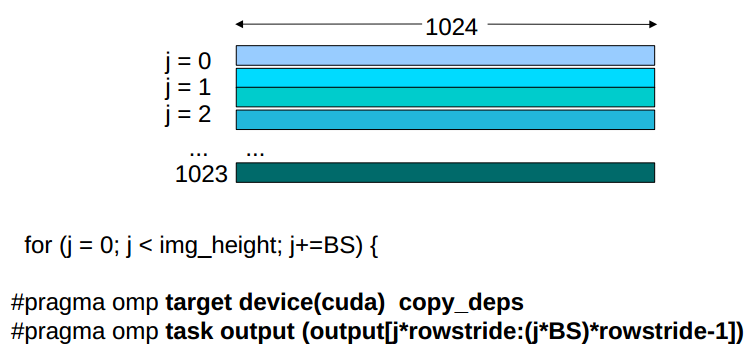# 3.2. Writing OmpSs programs¶

Following examples are written in C/C++ or Fortran using OmpSs as a programming model. With each example we provide simple explanations on how they are annotated and, in some cases, how they can be compiled (if a full example is provided).

## 3.2.1. Data Management¶

### 3.2.1.1. Reusing device data among same device kernel executions¶

Although memory management is completely done by the runtime system, in some cases we can assume a predefined behaviour. This is the case of the following Fortran example using an OpenCL kernel. If we assume runtime is using a `write-back` cache policy we can also determine that second kernel call will not imply any data movement.

kernel_1.cl:

```__kernel void vec_sum(int n, __global int* a, __global int* b, __global int* res)
{
const int idx = get_global_id(0);

if (idx < n) res[idx] = a[idx] + b[idx];
}
```

test_1.f90:

```! NOTE: Assuming write-back cache policy

SUBROUTINE INITIALIZE(N, VEC1, VEC2, RESULTS)
IMPLICIT NONE
INTEGER :: N
INTEGER :: VEC1(N), VEC2(N), RESULTS(N), I
DO I=1,N
VEC1(I) = I
VEC2(I) = N+1-I
RESULTS(I) = -1
END DO
END SUBROUTINE INITIALIZE

PROGRAM P
IMPLICIT NONE
INTERFACE
!\$OMP TARGET DEVICE(OPENCL) NDRANGE(1, N, 128) FILE(kernel_1.cl) COPY_DEPS
!\$OMP TASK IN(A, B) OUT(RES)
SUBROUTINE VEC_SUM(N, A, B, RES)
IMPLICIT NONE
INTEGER, VALUE :: N
INTEGER :: A(N), B(N), RES(N)
END SUBROUTINE VEC_SUM
END INTERFACE
INTEGER, PARAMETER :: N  = 20
INTEGER :: VEC1(N), VEC2(N), RESULTS(N), I

CALL INITIALIZE(N, VEC1, VEC2, RESULTS)

CALL VEC_SUM(N, VEC1, VEC2, RESULTS)
!  The vectors VEC1 and VEC2 are sent to the GPU. The input transfers at this
!   point are: 2 x ( 20 x sizeof(INTEGER)) = 2 x (20 x 4) = 160 B.

CALL VEC_SUM(N, VEC1, RESULTS, RESULTS)
!  All the input data is already in the GPU. We don't need to send
!   anything.

!  At this point we copy out from the GPU the computed values of RESULTS
!   and remove all the data from the GPU

! print the final vector's values
PRINT *, "RESULTS: ", RESULTS
END PROGRAM P

!  Expected IN/OUT transfers:
!   IN = 160B
!   OUT = 80B
```

Compile with:

```oclmfc -o test_1 test_1.f90 kernel_1.cl --ompss
```

### 3.2.1.2. Forcing data back using a taskwait¶

In this example, we need to copy back the data in between the two kernel calls. We force this copy back using a `taskwait`. Note that we are assuming `write-back` cache policy.

kernel_2.cl:

```__kernel void vec_sum(int n, __global int* a, __global int* b, __global int* res)
{
const int idx = get_global_id(0);

if (idx < n) res[idx] = a[idx] + b[idx];
}
```

test_2.f90:

```! NOTE: Assuming write-back cache policy

SUBROUTINE INITIALIZE(N, VEC1, VEC2, RESULTS)
IMPLICIT NONE
INTEGER :: N
INTEGER :: VEC1(N), VEC2(N), RESULTS(N), I
DO I=1,N
VEC1(I) = I
VEC2(I) = N+1-I
RESULTS(I) = -1
END DO
END SUBROUTINE INITIALIZE

PROGRAM P
IMPLICIT NONE
INTERFACE
!\$OMP TARGET DEVICE(OPENCL) NDRANGE(1, N, 128) FILE(kernel_2.cl) COPY_DEPS
!\$OMP TASK IN(A, B) OUT(RES)
SUBROUTINE VEC_SUM(N, A, B, RES)
IMPLICIT NONE
INTEGER, VALUE :: N
INTEGER :: A(N), B(N), RES(N)
END SUBROUTINE VEC_SUM
END INTERFACE
INTEGER, PARAMETER :: N  = 20
INTEGER :: VEC1(N), VEC2(N), RESULTS(N), I

CALL INITIALIZE(N, VEC1, VEC2, RESULTS)

CALL VEC_SUM(N, VEC1, VEC2, RESULTS)
!  The vectors VEC1 and VEC2 are sent to the GPU. The input transfers at this
!   point are: 2 x ( 20 x sizeof(INTEGER)) = 2 x (20 x 4) = 160 B.

!  At this point we copy out from the GPU the computed values of RESULT
!   and remove all the data from the GPU

PRINT *, "PARTIAL RESULTS: ", RESULTS

CALL VEC_SUM(N, VEC1, RESULTS, RESULTS)
!  The vectors VEC1 and RESULT are sent to the GPU. The input transfers at this
!   point are: 2 x ( 20 x sizeof(INTEGER)) = 2 x (20 x 4) = 160 B.

!  At this point we copy out from the GPU the computed values of RESULT
!   and remove all the data from the GPU

! print the final vector's values
PRINT *, "RESULTS: ", RESULTS
END PROGRAM P

!  Expected IN/OUT transfers:
!   IN = 320B
!   OUT = 160B
```

Compile with:

```oclmfc -o test_2 test_2.f90 kernel_2.cl --ompss
```

### 3.2.1.3. Forcing data back using a task¶

This example is similar to the example 1.2 but instead of using a `taskwait` to force the copy back, we use a task with copies. Note that we are assuming `write-back` cache policy.

kernel_3.cl:

```__kernel void vec_sum(int n, __global int* a, __global int* b, __global int* res)
{
const int idx = get_global_id(0);

if (idx < n) res[idx] = a[idx] + b[idx];
}
```

test_3.f90:

```! NOTE: Assuming write-back cache policy

SUBROUTINE INITIALIZE(N, VEC1, VEC2, RESULTS)
IMPLICIT NONE
INTEGER :: N
INTEGER :: VEC1(N), VEC2(N), RESULTS(N), I
DO I=1,N
VEC1(I) = I
VEC2(I) = N+1-I
RESULTS(I) = -1
END DO
END SUBROUTINE INITIALIZE

PROGRAM P
IMPLICIT NONE
INTERFACE
!\$OMP TARGET DEVICE(OPENCL) NDRANGE(1, N, 128) FILE(kernel_3.cl) COPY_DEPS
!\$OMP TASK IN(A, B) OUT(RES)
SUBROUTINE VEC_SUM(N, A, B, RES)
IMPLICIT NONE
INTEGER, VALUE :: N
INTEGER :: A(N), B(N), RES(N)
END SUBROUTINE VEC_SUM

!\$OMP TARGET DEVICE(SMP) COPY_DEPS
SUBROUTINE PRINT_BUFF(N, BUFF)
IMPLICIT NONE
INTEGER, VALUE :: N
INTEGER :: BUFF(N)
END SUBROUTINE VEC_SUM
END INTERFACE

INTEGER, PARAMETER :: N  = 20
INTEGER :: VEC1(N), VEC2(N), RESULTS(N), I

CALL INITIALIZE(N, VEC1, VEC2, RESULTS)

CALL VEC_SUM(N, VEC1, VEC2, RESULTS)
!  The vectors VEC1 and VEC2 are sent to the GPU. The input transfers at this
!   point are: 2 x ( 20 x sizeof(INTEGER)) = 2 x (20 x 4) = 160 B.

CALL PRINT_BUFF(N, RESULTS)
!  The vector RESULTS is copied from the GPU to the CPU. The copy of this vector in
!   the memory of the GPU is not removed because the task 'PRINT_BUFF' does not modify it.
!   Output transfers: 80B.
!   VEC1 and VEC2 are still in the GPU.

CALL VEC_SUM(N, VEC1, RESULTS, RESULTS)
!  The vectors VEC1 and RESULTS are already in the GPU. Do not copy anything.

CALL PRINT_BUFF(N, RESULTS)
!  The vector RESULTS is copied from the GPU to the CPU. The copy of this vector in
!   the memory of the GPU is not removed because the task 'PRINT_BUFF' does not it.
!   Output transfers: 80B.
!   VEC1 and VEC2 are still in the GPU.

!  At this point we remove all the data from the GPU. The right values of the vector RESULTS are
!   already in the memory of the CPU, then we don't need to copy anything from the GPU.

END PROGRAM P

SUBROUTINE PRINT_BUFF(N, BUFF)
IMPLICIT NONE
INTEGER, VALUE :: N
INTEGER :: BUFF(N)

PRINT *, "BUFF: ", BUFF
END SUBROUTINE VEC_SUM

!  Expected IN/OUT transfers:
!   IN = 160B
!   OUT = 160B
```

Compile with:

```oclmfc -o test_3 test_3.f90 kernel_3.cl --ompss
```

## 3.2.2. Application’s kernels¶

### 3.2.2.1. BlackScholes¶

This benchmark computes the pricing of European-style options. Its kernel has 6 input arrays, and a single output. Offloading is done by means of the following code:

```for (i=0; i<array_size; i+= chunk_size ) {
int elements;
unsigned int * cpf;
elements = min(i+chunk_size, array_size ) - i;
cpf = cpflag;
#pragma omp target device(cuda) copy_in( \
cpf   [i;elements], \
S0    [i;elements], \
K     [i;elements], \
r     [i;elements], \
sigma [i;elements], \
T     [i;elements]) \
#pragma omp task firstprivate(local_work_group_size, i)
{
dim3 dimBlock(local_work_group_size, 1 , 1);
dim3 dimGrid(elements / local_work_group_size, 1 , 1 );
cuda_bsop <<<dimGrid, dimBlock>>> (&cpf[i], &S0[i], &K[i],

&r[i], &sigma[i], &T[i], &answer[i]);
}
}
```

Following image shows graphically the annotations used to offload tasks to the GPUs available. Data arrays annotated with the copy_in clause are automatically transferred by the Nanos++ runtime system onto the GPU global memory. After the CUDA kernel has been executed, the copy_out clause indicates to the runtime system that the results written by the GPU onto the output array should be synchronized onto the host memory. This is done at the latest when the host program encounters the `taskwait` directive.### 3.2.2.2. Perlin Noise¶

This benchmark generates an image consisting of noise, useful to be applied to gaming applications, in order to provide realistic effects. The application has a single output array, with the generated image. Annotations are shown here:

```for (j = 0; j < img_height; j+=BS) {
// Each task writes BS rows of the image
#pragma omp target device(cuda) copy_deps
#pragma omp task output (output[j*rowstride:(j+BS)*rowstride-1])
{
dim3 dimBlock;
dim3 dimGrid;
dimBlock.x = (img_width < BSx) ? img_width : BSx;
dimBlock.y = (BS < BSy) ? BS : BSy;
dimBlock.z = 1;
dimGrid.x = img_width/dimBlock.x;
dimGrid.y = BS/dimBlock.y;
dimGrid.z = 1;

cuda_perlin <<<dimGrid, dimBlock>>> (&output[j*rowstride], time, j, rowstride);
}
}
#pragma omp taskwait noflush
```

In this example, the `noflush` clause eliminates the need for the data synchronization implied by the `taskwait` directive. This is useful when the programmer knows that the next task that will be accessing this result will also be executed in the GPUs, and the host program does not need to access it. The runtime system ensures in this case that the data is consistent across GPUs.

Following image shows the graphical representation of the data, and the way annotations split it across tasks.### 3.2.2.3. N-Body¶

This benchmark implements the gravitational forces among a set of particles. It works with an input array (this_particle_array), and an output array (output_array). Mass, velocities, and positions of the particles are kept updated alternatively in each array by means of a pointer exchange. The annotated code is shown here:

```void Particle_array_calculate_forces_cuda ( int number_of_particles,
Particle this_particle_array[number_of_particles],
Particle output_array[number_of_particles],
float time_interval )
{
const int bs = number_of_particles/8;